American Journal of Applied Mathematics
Volume 4, Issue 5, October 2016, Pages: 252-257

Parameter Estimation of a DC Motor-Gear-Alternator (MGA) System via Step Response Methodology

Wesley Koech1, *, Titus Rotich2, Fredrick Nyamwala1, Samwel Rotich1

1Department of Mathematics and Physics, School of Biological and Physical Sciences, Moi University, Eldoret, Kenya

2Department of Centre for Teacher Education, School of Education, Moi University, Eldoret, Kenya

Email address: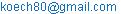(W. Koech)

*Corresponding author

To cite this article:

Wesley Koech, Samwel Rotich, Titus Rotich, Fredrick Nyamwala. Parameter Estimation of a DC Motor-Gear-Alternator (MGA) System via Step Response Methodology. American Journal of Applied Mathematics. Vol. 4, No. 5, 2016, pp. 252-257. doi: 10.11648/j.ajam.20160405.17

Received: September 16, 2016; Accepted: October 2, 2016; Published: October 27, 2016

Abstract: Mathematical models and their parameters are essential when designing controllers because they allow the designer to predict the closed loop behavior of the system. An accurate method for estimating the DC Motor-Gear-Alternator (MGA) system parameters is needed before constructing the reliable model. This paper proposed a new method of parameter estimation using Matlab/Simulink parameter estimation tool via Step Response Methodology. Optimization algorithms including the nonlinear least square, Gradient Descent, Simplex Search and Pattern Search are discussed. Simulink Design Optimization automatically estimated parameters of the MGA model from measured input-output data.

Keywords: Estimation, Step Response, DC Motor, Alternator, Simulink, Optimization

Contents

1. Introduction

Errors in parameter values can lead to poor control and instability. Therefore, accuracy and adequacy of parameters identification are too major modeling issues that always have to be dealt with . The main purpose of most System parameter estimation is to develop a mathematical model that fully describes an underlying physical system which can be used to predict both the response of the system to disturbance and the design advantage which might be obtained from modification of the system . This mathematical model can be used to explain the behavior of the system and to predict its response to various inputs at different conditions . Methods to estimate process model parameters from both open loop and closed loop step responses was proposed . A number of methods for system parameter estimation [4-11] can be applied to MGA model identification. In particular, various methods have been used to estimate parameter of a model; that is, [5,7,8] used the algebraic identification techniques,  used a recursive least-squares parameter estimation algorithm for non-uniformly sampled multirate systems,  used an inverse problem theory and methods for model parameter estimation,  applied an algorithm for least-squares estimation of nonlinear parameters and  designed optimization of parameters using response surface methodology with central composite.

1.1. Open-Loop System

The physical model for the DC Motor-Gear-Alternator used in this study is shown in Figure 1 .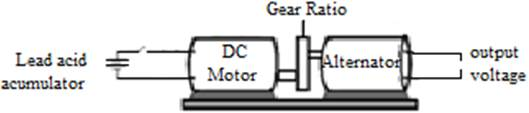Figure 1. Physical model for the MGA.

The motor and the alternator of the physical system are assembled as shown in Figure 1. The gear unit is introduced between the motor and the alternator as an amplifying system. Since motors with low rotating speed and high torque are used often in control systems, the gear train is often adopted to increase the speed and reduce the torque.

1.2. Schematic Representation of the System

The diagram shown in Figure 2 is a schematic representation for the MGA model .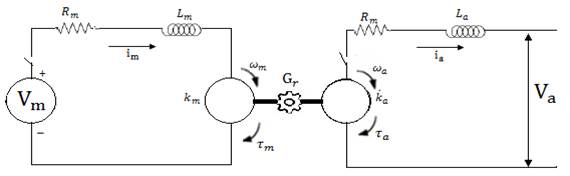Figure 2. Schematic Representation of the MGA model.

1.3. Simulink Model of the MGA System

The Simulink model for the system  is shown in Figure 3.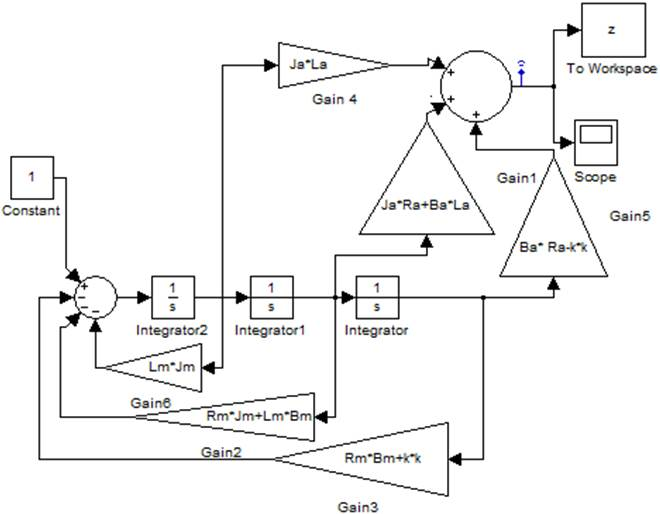Figure 3. Simulink Model of the MGA System.

The corresponding transfer function is given by equation (1)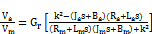(1)

2. Parameter Identification

Parameter identification we have used is the one outlined by . This method is built in Simulink Parameter Estimation software in MATLAB for the parameters identification of the MGA model. Simulink Parameter Estimation soft-ware is a Simulink-based product for estimating and calibrating model parameters from experimental data. This product supports the following types of estimation:

Transient Estimation: Estimate parameters by comparing model output to the experimental data for a given input.

Initial Condition Estimation: Estimate the initial conditions of states using experimental data.

Adaptive Lookup Tables: Estimate the table values at the prescribed break-points by using measurements from the physical system.

Simulink Parameter Estimation software provides the tools used to:

(a)    Set up the problem.

(b)    Specify which model parameters to estimate.

(c)    Import and prepare the experimental data for parameter estimation (or pre-process).

(d)    View the estimation progress.

(e)    Validate the estimation results based on plots of measured versus simulated data and residuals.

2.1. Simulink Parameter Estimation

Simulink Parameter Estimation software compares empirical data with data generated by a Simulink model. Using optimization techniques, the software estimates the parameter and (optionally) initial conditions of states such that a user-selected cost function is minimized. The cost function typically calculates a least-square error between the empirical and model data signals.

After building a Simulink model, the following steps are followed to configure and run parameter estimation:

(a)    Select Tools Parameter Estimation in Simulink model window. This opens the Control and Estimation Tools Manager, creates a new project, and adds an Estimation node to the workspace directory tree.

(b)    The input and output data set for estimating parameters of Simulink model are imported.

(c)    The parameters and initial conditions to be estimated are selected.

(d)    Configure the estimation itself, including cost functions and data views.

(e)    Run the estimation.

(f)     Check the results by examining the cost-function values, plots, or parameter values.

2.2. Description of the DC MGA System

The Simulink model under study is shown in Figure 3. The model consists of input port for acquiring input signal of motor voltage and the output port for acquiring the output signals of alternator voltage. The alternator consists of a spinning set of electrical windings called a rotor, a stationary set of windings called a stator, a rectifier assembly, a set of brushes to maintain electrical contact with the rotor. DC motor controls the frequency of the alternator. There are also Gears attached in between alternator and the DC motor to amplify the frequency when the DC motor is energized. The amount of rotation of the motor shaft is limited to gear ratio. Therefore, if a large gear ratio is used, the motor stops. Hence, we are modeling a system with gear ratio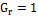which gives better numerical properties and easier notation. We know the model components as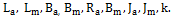However, the parameter values of the system are not known. Hence the parameters need to be estimated for a better fit.

We estimated these parameters as precisely as possible for our model to ascertain whether it is an accurate representation of the actual MGA system. When we applied a step voltage to the motor input, the alternator displays some voltage in response. However, if the model parameters do not match those of the step response, the model response will not match that of the actual system, either. Figure 6 shows the voltage response of our model using the initial parameter values in the model with a unit input voltage. Figure 5 show the required step response . It is obvious that there is need to estimate model parameters. This is where Simulink Parameter Estimation plays a crucial role. The software formulates parameter estimation as an optimization problem. The optimization problem solutions are the estimated parameter values. The data used for estimation are real data collected from experimental alternator .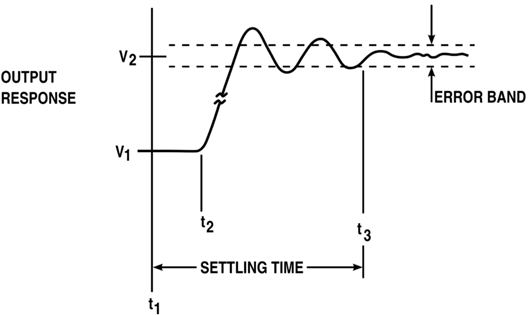Figure 4. Required Voltage Step Response.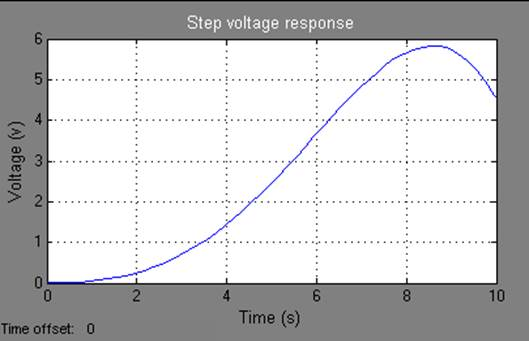Figure 5. Voltage Response.

3. Data Estimation

A new data set can be created by clicking on the "Transient Data" node and pressing the "New" button in the right-hand-side panel. These data sets can then be used for estimation and or validation. We have already defined three data sets. The first is used for parameter estimation and the remaining two for validating the response of the Simulink model with the estimated parameters. These data sets can be imported from various sources including MATLAB® variables, MAT files, Excel® files, or comma-separated-value files. Once we import the data, we can plot them to confirm that we have the right data sets in our estimation project.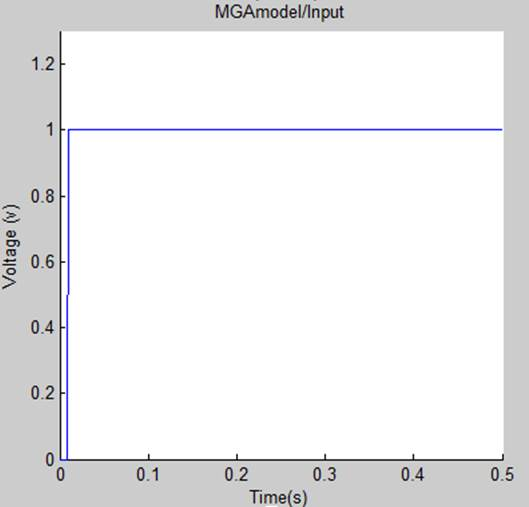Figure 6. Output Response of the MGA.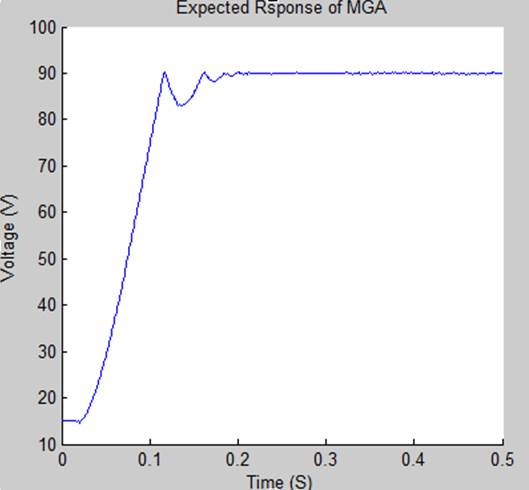Figure 7. Expected Response of the MGA.

We can see the simulation data does not match the measured data because the parameters are incorrect. Hence we used Simulink design optimization to automatically tune model parameters

3.1. Definition of Variables

The next step is to define the variables for the estimation. This establishes which parameters of the simulation can be adjusted, and any rules governing their values. The Estimation variables are selected by clicking on the "Variables" node and pressing the "Add" button. This opens a "Parameter Selection Dialog" from which we can select the model parameters that we desire to estimate.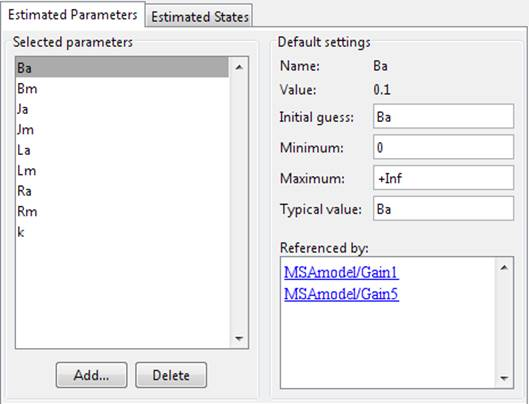Figure 8. Selecting Parameters for Estimation.

We have already added the nine unknown parameters in our model using the selection dialog. These parameters are the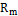Armature Resistance of the Motor,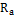Armature Resistance of alternator,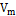Motor Voltage,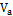Alternator Voltage,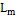Inductance of the motor,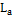Inductance of the alternator,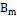Viscous friction coefficients of the motor,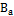Viscous friction coefficients of the alternator and the back e.m.f coefficient k. On the panel to the right of the list of parameters, you can set the initial guesses for the parameter values, and the minimum and maximum bounds on these values. Since we know from our physical insight that all of these parameters have positive values, we set their lower limits to zero. We can also select an initial value for the parameters.

3.2. The Estimation Task

In order to run estimation, we first need to create an "Estimation" node. This is done by clicking on the "Estimation node and pressing the "New" button in the right-hand-side panel. In our project, we have already created an estimation node called "New Estimation". We can click on this node to set up its various options. The first panel is where we select the data sets to be used in this estimation. It is possible to use one or more data sets at once in a given estimation. For this model, we used the data set called "Estimation Data". The next panel called "Parameters" is where we select which parameters to adjust in this estimation. Even though we selected nine parameters, in general, it is not necessary to estimate all of them at once. However, since our model is simple enough we will estimate all nine parameters.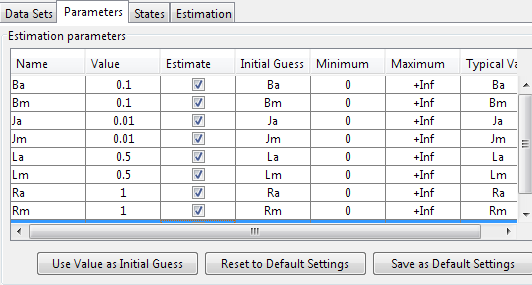Figure 9. Selecting Parameters to Adjust.

3.3. Viewing the Results

Four plot types are created to view the estimation results. The plot in Figure 10 shows the experimental data overlaid with the simulated data. The simulated data come from the model with the estimated parameters. The results of the estimation appear satisfactory as the estimated blue and green curve closely matches the measured results.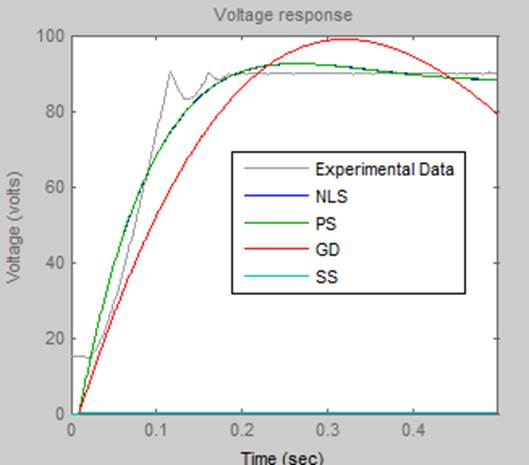Figure 10. Voltage Response for the Actual and Estimated Models.

We can see that as the parameters values improve, the simulation curves get closer to the experimental data. Figure 10 shows voltage response after 150 iterations. It is clear from these figures that simulation results based on identified parameters are consistent with actual results

We can also view how the parameters changed in the model. The plot in Figure 11 shows the trajectories of the parameters at each iteration of the estimation process. It is shown that the parameters settle to their final values as the estimation process converges to a solution.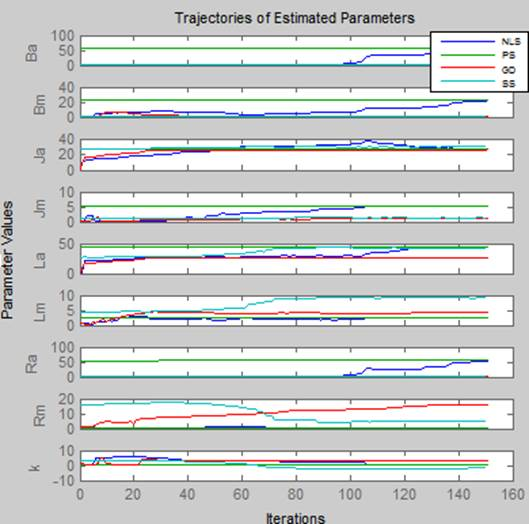Figure 11. Simulation Trajectories.

Table 1 shows us the results for the different algorithms used. It can be observed that the parameter values of NLS and PS methods are approximately the same and closer to the expected values.

Table 1. Summary of parameter estimation results of the four methods.

 Parameters NLS PS GD SS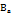57.60 57.10 1.90 0.471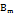22.00 23.00 0.00 0.252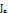26.91 27.00 26.11 0.019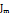5.07 5.07 0.93 -0.032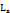44.10 44.04 26.80 3.963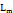2.67 2.67 4.30 -1.844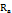54.34 57.22 1.53 -0.144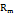0.09 0.09 15.53 2.2780.70 0.01 3.10 -2.144

The transfer function of the MGA model is needed for studying the state of our system and applying the controlling techniques on it. The transfer function for MGA model is obtained by substituting parameter values of NLS or PS of Table 1 into equation 1 to obtain transfer functions as,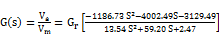(2)

4. Conclusion

The Nonlinear Least Square and Pattern Search methods are the best algorithms which can be used to estimate the parameters of the MGA system because of the performance and the accurate in results and can be automated in MATLAB. The choice of algorithm depends on the nature of the system. The Simplex Search option cannot be used to estimate parameters or states with lower and upper bounds. The goodness of step response is that functions are easy to derive and experiments are easy to implement. Though similar responses are possible from different models and that steps may not be sufficiently small to avoid non-linear behavior.

Future Work

There is work underway to determine the stability of the model for practical use.

Competing Interests

Authors have declared that no competing interests exist.

References

1. Byrne, B.M., Structural equation modeling with AMOS: Basic concepts, applications, and programming. 2013: Routledge.
2. Åström, K.J. and B. Wittenmark, Adaptive control. 2013: Courier Corporation.
3. Basilio, J.C. and M.V. Moreira, State-space parameter identification in a second control laboratory. Education, IEEE Transactions on, 2004. 47(2): p. 204-210.
4. Ahmed, S., B. Huang, and S.L. Shah, Novel identification method from step response. Control Engineering Practice, 2007. 15(5): p. 545-556.
5. Fliess, M. and H. Sira-Ramirez, Closed-loop parametric identification for continuous-time linear systems via new algebraic techniques, in Identification of Continuous-time Models from sampled Data. 2008, Springer. p. 363-391.
6. Liu, Y., L. Xie, and F. Ding, An auxiliary model based on a recursive least-squares parameter estimation algorithm for non-uniformly sampled multirate systems. Proceedings of the Institution of Mechanical Engineers, Part I: Journal of Systems and Control Engineering, 2009. 223(4): p. 445-454.
7. Mamani, G., et al. Open-loop algebraic identification method for a DC motor. in Control Conference (ECC), 2007 European. 2007. IEEE.
8. Mamani, G., J. Becedas, and V. Feliu-Batlle. On-Line Fast Algebraic Parameter and State Estimation for a DC Motor Applied to Adaptive Control. in Proceedings of the World Congress on Engineering. 2008.
9. Plett, G.L., Extended Kalman filtering for battery management systems of LiPB-based HEV battery packs: Part 3. State and parameter estimation. Journal of Power sources, 2004. 134(2): p. 277-292.
10. Tarantola, A., Inverse problem theory and methods for model parameter estimation. 2005: siam.
11. Kumar, P.R. and P. Varaiya, Stochastic systems: Estimation, identification, and adaptive control. Vol. 75. 2015: SIAM.
12. Asfaram, A., et al., Removal of basic dye Auramine-O by ZnS: Cu nanoparticles loaded on activated carbon: optimization of parameters using response surface methodology with central composite design. RSC Advances, 2015. 5(24): p. 18438-18450.
13. Koech, W., et al., Dynamic Model of a DC Motor-gear-alternator (MGA) System. Asian Research Journal of Mathematics, 2016. 1(4): p. 16.
14. Salah, M.S., Parameters identification of a permanent magnet DC motor. 2009, The Islamic University of Gaza.
15. Fink, A., How to conduct surveys: A step-by-step guide. 2015: Sage Publications.
16. Rey, G., et al., Performance analysis, model development and validation with experimental data of an ICE-based micro-CCHP system. Applied Thermal Engineering, 2015. 76: p. 233-244.

 Contents 1. 1.1. 1.2. 1.3. 2. 2.1. 2.2. 3. 3.1. 3.2. 3.3. 4.
Article ToolsAbstractPDF(517K)
Follow on us
PUBLICATION SERVICE
JOIN US
RESOURCES
SPECIAL SERVICES
ADDRESS
Science Publishing Group
548 FASHION AVENUE
NEW YORK, NY 10018
U.S.A.
Tel: (001)347-688-8931